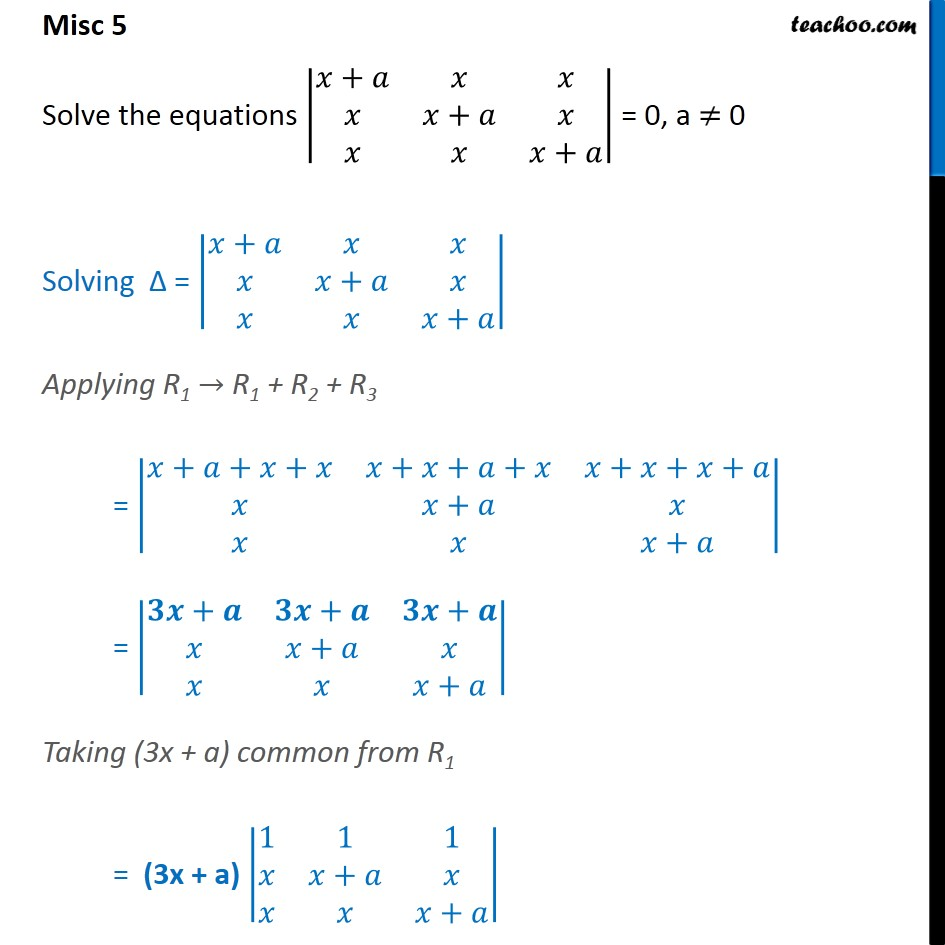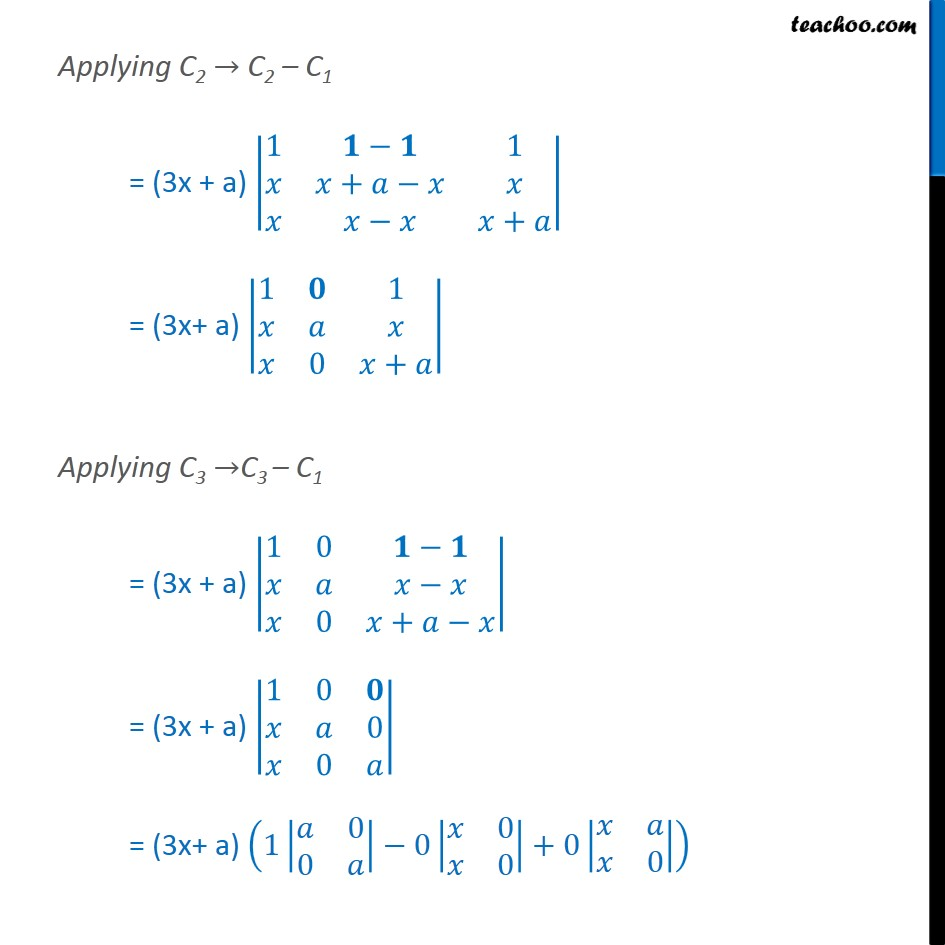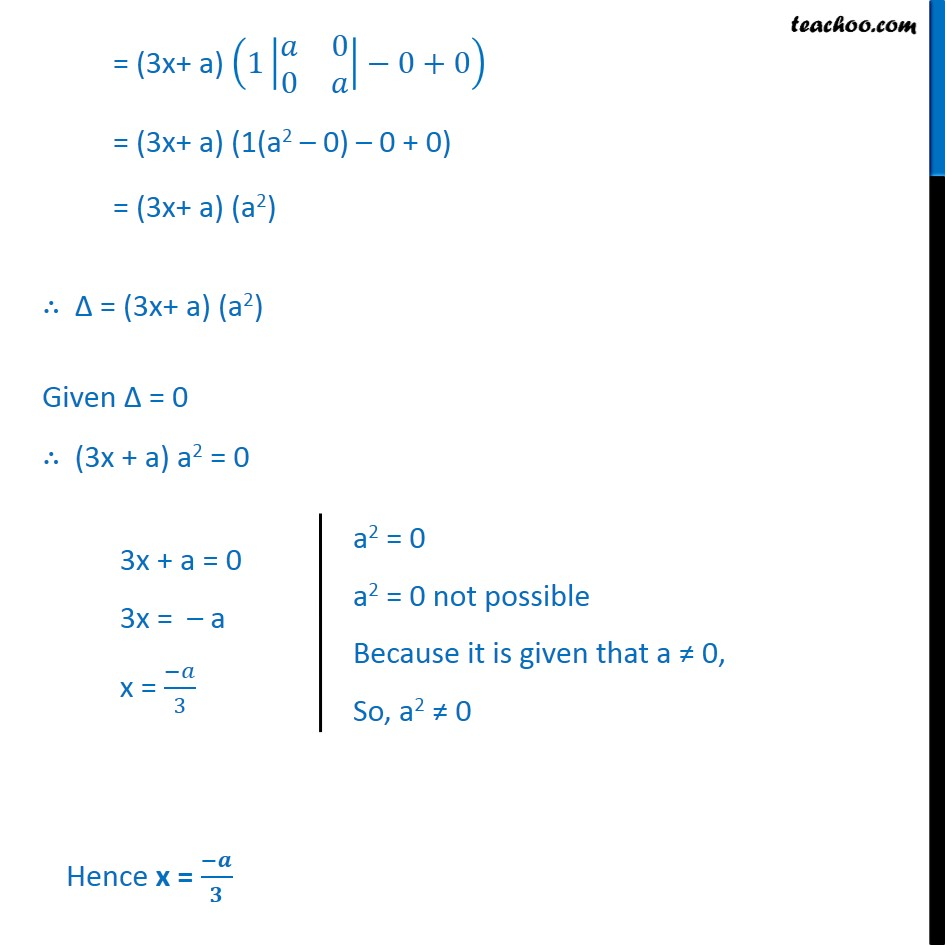Miscellaneous

Chapter 4 Class 12 Determinants
Serial order wiseLearn in your speed, with individual attention - Teachoo Maths 1-on-1 Class

### Transcript

Question 3 Solve the equations 𝑥+𝑎﷮𝑥﷮𝑥﷮𝑥﷮𝑥+𝑎﷮𝑥﷮𝑥﷮𝑥﷮𝑥+𝑎﷯﷯ = 0, a ≠ 0 Solving ∆ = 𝑥+𝑎﷮𝑥﷮𝑥﷮𝑥﷮𝑥+𝑎﷮𝑥﷮𝑥﷮𝑥﷮𝑥+𝑎﷯﷯ Applying R1 → R1 + R2 + R3 = 𝑥+𝑎+𝑥+𝑥﷮𝑥+𝑥+𝑎+𝑥﷮𝑥+𝑥+𝑥+𝑎﷮𝑥﷮𝑥+𝑎﷮𝑥﷮𝑥﷮𝑥﷮𝑥+𝑎﷯﷯ = 𝟑𝒙+𝒂﷮𝟑𝒙+𝒂﷮𝟑𝒙+𝒂﷮𝑥﷮𝑥+𝑎﷮𝑥﷮𝑥﷮𝑥﷮𝑥+𝑎﷯﷯ Taking (3x + a) common from R1 = (3x + a) 1﷮1﷮1﷮𝑥﷮𝑥+𝑎﷮𝑥﷮𝑥﷮𝑥﷮𝑥+𝑎﷯﷯ Applying C2 → C2 – C1 = (3x + a) 1﷮𝟏−𝟏﷮1﷮𝑥﷮𝑥+𝑎−𝑥﷮𝑥﷮𝑥﷮𝑥−𝑥﷮𝑥+𝑎﷯﷯ = (3x+ a) 1﷮𝟎﷮1﷮𝑥﷮𝑎﷮𝑥﷮𝑥﷮0﷮𝑥+𝑎﷯﷯ Applying C3 →C3 – C1 = (3x + a) 1﷮0﷮𝟏−𝟏﷮𝑥﷮𝑎﷮𝑥−𝑥﷮𝑥﷮0﷮𝑥+𝑎−𝑥﷯﷯ = (3x + a) 1﷮0﷮𝟎﷮𝑥﷮𝑎﷮0﷮𝑥﷮0﷮𝑎﷯﷯ = (3x+ a) 1 𝑎﷮0﷮0﷮𝑎﷯﷯−0 𝑥﷮0﷮𝑥﷮0﷯﷯+0 𝑥﷮𝑎﷮𝑥﷮0﷯﷯﷯ = (3x+ a) 1 𝑎﷮0﷮0﷮𝑎﷯﷯−0+0﷯ = (3x+ a) (1(a2 – 0) – 0 + 0) = (3x+ a) (a2) ∴ ∆ = (3x+ a) (a2) Given ∆ = 0 ∴ (3x + a) a2 = 0